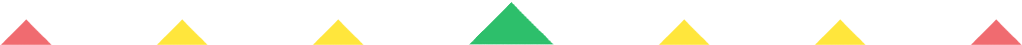Player 1
vs
Player 2
Your History
0:00
Opponent history
x
xCorrect
0
Wrong
0
Correct!
Wrong!
Help Sheet for

• 0 x 2 = 0
• 1 x 2 = 2
• 2 x 2 = 4
• 3 x 2 = 6
• 4 x 2 = 8
• 5 x 2 = 10
• 6 x 2 = 12
• 7 x 2 = 14
• 8 x 2 = 16
• 9 x 2 = 18
• 10 x 2 = 20
• 11 x 2 = 22
• 12 x 2 = 24

• 0 x 3 = 0
• 1 x 3 = 3
• 2 x 3 = 6
• 3 x 3 = 9
• 4 x 3 = 12
• 5 x 3 = 15
• 6 x 3 = 18
• 7 x 3 = 21
• 8 x 3 = 24
• 9 x 3 = 27
• 10 x 3 = 30
• 11 x 3 = 33
• 12 x 3 = 36

• 0 x 4 = 0
• 1 x 4 = 4
• 2 x 4 = 8
• 3 x 4 = 12
• 4 x 4 = 16
• 5 x 4 = 20
• 6 x 4 = 24
• 7 x 4 = 28
• 8 x 4 = 32
• 9 x 4 = 36
• 10 x 4 = 40
• 11 x 4 = 44
• 12 x 4 = 48

• 0 x 5 = 0
• 1 x 5 = 5
• 2 x 5 = 10
• 3 x 5 = 15
• 4 x 5 = 20
• 5 x 5 = 25
• 6 x 5 = 30
• 7 x 5 = 35
• 8 x 5 = 40
• 9 x 5 = 45
• 10 x 5 = 50
• 11 x 5 = 55
• 12 x 5 = 60